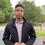# The basics for a programmer

1. The Peano axioms

The entire formalization of arithmetic is based on five fundamental axioms, called Peano axioms, which define properties of natural numbers. These axioms are -

(i) 0 is a natural number (ii) Every natural number has a successor, which is also a natural number (iii) 0 is not the successor of any natural number (iv) Different natural numbers have different successors (v) If a set contains the number 0 and it also contains the successor of every number in S, then S contains every natural number.

The fifth axiom is also popularly known as "principal of mathematical induction"

Being extremely basic, we would rarely need them directly, unless we want to prove every theorem in arithmetic from the first principles. But being the building blocks of arithmetic, these axioms are worth knowing.

1. Fundamental Theorem of Arithmetic and the Division Algorithm

As the name rightly says, this theorem lies at the heart of all the concepts in number theory. The fundamental theorem of arithmetic states that any integer greater than 1 can be written as a product of prime numbers in a unique way (up to the ordering of prime factors in the product). For example, 18 = 2 X 32, 1755 = 33 X 5 X 13. This theorem plays very important role in almost every number theoretic algorithm, like finding prime factors, finding GCD, finding sum of divisors of a number etc to mention a few. Proving this theorem is easy - in fact, it emerges out as a corollary to the Euclid's first theorem (discussed below).

The division algorithm states that given two integers a,b (b != 0) there exist two unique integers q and r such that

a = bq + r, 0 <= r < b

q is typically called quotient, whereas r is called remainder. If r = 0, we say that b divides a, and denote it as b|a.

Further Reading: wikipedia, walfram , divisibility , division algorithm

1. Euclid's Theorems

The two important theorems, called "Euclid's first theorem (Or Euclid's lemma)" and "Euclid's second theorem (usually simply referred as "Euclid's theorem") are as follows:

First theorem: p | ab => p|a or p | b. A direct consequence of this is the fundamental theorem of arithmetic. Second theorem : There are infinitely many primes. There are many simple proofs for this.

While it is true that there are infinitely many primes, it should also be remembered that there are arbitarily large gap between prime numbers. In other words, it is always possible to get a sequence of n consecutive composite numbers, given n.

Further Reading: Euclid's Theorem, Euclid's Lemma, walfram

1. GCD, LCM, Bezout's identity

The most common algorithm for finding the greatest common divisor of two numbers is the Euclid's algorithm. This is an extremely efficient algorithm, as the number of steps required in this algorithm is at most 5 times the number of digits of the smaller number. GCD is typically denoted using round brackets - (a,b) denotes the gcd of a and b. Similarly LCM is denoted using square brackets - [a,b] denotes the lcm of a and b.

The numbers a and b are called coprimes iff (a,b) = 1 , i.e. iff [a,b] = ab.

If gcd(a,b) = d then (a/d, b/d) = 1.

GCD and LCM are related by a very simple equation: (a,b) * [a,b] = ab. This gives a very fast way to calculate LCM of two numbers.

The bezout's identity states that if d = (a,b) then there always exist integers x and y such that ax+by = d. (Of course, the theory of linear diophantine equations assures existance of infinitely many solutions, if one exists). It is also worth noting that k=d is the smallest positive integer for which ax+by = k has a solution with integral x and y.

Given a,b, Finding x and y, such that ax+by = d is done by extended Euclid's algorithm, which can be implemented in recursive as well as iterative styles.

Further Reading: GCD, Bezout's Identity, Euclid's Algorithm, Extended Euclid's Algorithm

SourceNote by Mardokay Mosazghi
7 years, 4 months ago

This discussion board is a place to discuss our Daily Challenges and the math and science related to those challenges. Explanations are more than just a solution — they should explain the steps and thinking strategies that you used to obtain the solution. Comments should further the discussion of math and science.

When posting on Brilliant:

• Use the emojis to react to an explanation, whether you're congratulating a job well done , or just really confused .
• Ask specific questions about the challenge or the steps in somebody's explanation. Well-posed questions can add a lot to the discussion, but posting "I don't understand!" doesn't help anyone.
• Try to contribute something new to the discussion, whether it is an extension, generalization or other idea related to the challenge.

MarkdownAppears as
*italics* or _italics_ italics
**bold** or __bold__ bold
- bulleted- list
• bulleted
• list
1. numbered2. list
1. numbered
2. list
Note: you must add a full line of space before and after lists for them to show up correctly
paragraph 1paragraph 2

paragraph 1

paragraph 2

[example link](https://brilliant.org)example link
> This is a quote
This is a quote
    # I indented these lines
# 4 spaces, and now they show
# up as a code block.

print "hello world"
# I indented these lines
# 4 spaces, and now they show
# up as a code block.

print "hello world"
MathAppears as
Remember to wrap math in $$ ... $$ or $ ... $ to ensure proper formatting.
2 \times 3 $2 \times 3$
2^{34} $2^{34}$
a_{i-1} $a_{i-1}$
\frac{2}{3} $\frac{2}{3}$
\sqrt{2} $\sqrt{2}$
\sum_{i=1}^3 $\sum_{i=1}^3$
\sin \theta $\sin \theta$
\boxed{123} $\boxed{123}$

Sort by:

Nice note, @Mardokay Mosazghi

- 6 years, 8 months ago

I will try to do some latex editing for your note. Keep writing. :)

- 6 years, 8 months ago